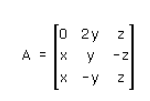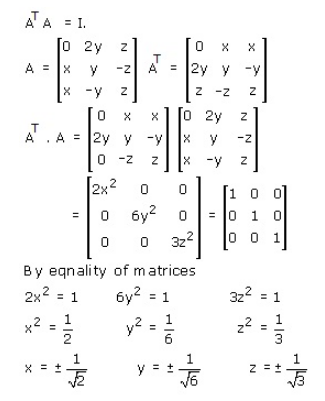# Find the values of x, y, z if the matrix product AT A = I where

Find the values of x, y, z if the matrix product AT A = I where